NEET  >  31 Year NEET Previous Year Questions: Work, Energy & Power - 1

# 31 Year NEET Previous Year Questions: Work, Energy & Power - 1

Test Description

## 23 Questions MCQ Test Physics Class 11 | 31 Year NEET Previous Year Questions: Work, Energy & Power - 1

31 Year NEET Previous Year Questions: Work, Energy & Power - 1 for NEET 2023 is part of Physics Class 11 preparation. The 31 Year NEET Previous Year Questions: Work, Energy & Power - 1 questions and answers have been prepared according to the NEET exam syllabus.The 31 Year NEET Previous Year Questions: Work, Energy & Power - 1 MCQs are made for NEET 2023 Exam. Find important definitions, questions, notes, meanings, examples, exercises, MCQs and online tests for 31 Year NEET Previous Year Questions: Work, Energy & Power - 1 below.
Solutions of 31 Year NEET Previous Year Questions: Work, Energy & Power - 1 questions in English are available as part of our Physics Class 11 for NEET & 31 Year NEET Previous Year Questions: Work, Energy & Power - 1 solutions in Hindi for Physics Class 11 course. Download more important topics, notes, lectures and mock test series for NEET Exam by signing up for free. Attempt 31 Year NEET Previous Year Questions: Work, Energy & Power - 1 | 23 questions in 45 minutes | Mock test for NEET preparation | Free important questions MCQ to study Physics Class 11 for NEET Exam | Download free PDF with solutions
 1 Crore+ students have signed up on EduRev. Have you?
31 Year NEET Previous Year Questions: Work, Energy & Power - 1 - Question 1

### A 0.5 kg ball moving with speed of 12 m/s strikes a hard wall at an angle of 30° with the wall. It is reflected with the same speed and at the same angle. If the ball is in contact with the wall for 0.25 seconds, the average force acting on the wall is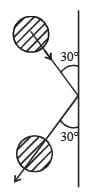Detailed Solution for 31 Year NEET Previous Year Questions: Work, Energy & Power - 1 - Question 1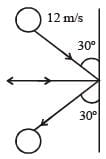Resolving the velocities in vertical and horizontal directions, resolved parts of first velocity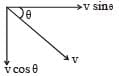v cosθ perpendicular to the wall and v sinθ parallel to the wall. In the second case, they are –v sinθ & v cosθ respectively. Here, –ve sign is because direction is opposite to the earlier ones. So we see a net change in velocity perpendicular to way = v sinθ – (–v sinθ) = 2v sinθ
This change has occured in 0.25 sec, so, rate  of change of velocity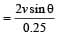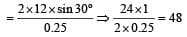Thus, acceleration a = 48 m/sec2

Force applied = m . a = 0.5 × 48 = 24 N

31 Year NEET Previous Year Questions: Work, Energy & Power - 1 - Question 2

### A vertical spring with force constant k is fixed on a table. A ball of mass m at a height h above the free upper end of the spring falls vertically on the spring so that the spring is compressed by a distance d. The net work done in the process is  

Detailed Solution for 31 Year NEET Previous Year Questions: Work, Energy & Power - 1 - Question 2

Gravitational potential energy of ball gets converted into elastic potential energy of the spring.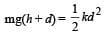Net work done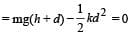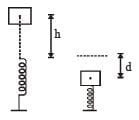31 Year NEET Previous Year Questions: Work, Energy & Power - 1 - Question 3

### Water falls from a height of 60 m at the rate of 15 kg/s to operate a turbine. The losses due to frictional force are 10% of energy. How much power is generated by the turbine?( g = 10 m/s2)

Detailed Solution for 31 Year NEET Previous Year Questions: Work, Energy & Power - 1 - Question 3

Given, h = 60m, g = 10 ms–2, Rate of flow of water = 15 kg/s

∴ Power of the falling water = 15 kgs–1 × 10 ms–2 × 60 m = 900 watt.
Loss in energy due to friction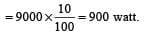∴ Power generated by the turbine = ( 9000 – 900) watt = 8100 watt = 8.1 kW

31 Year NEET Previous Year Questions: Work, Energy & Power - 1 - Question 4

A shell of mass 200 gm is ejected from a gun of mass 4 kg by an explosion that generates 1.05 kJ of energy. The initial velocity of the shell is:

Detailed Solution for 31 Year NEET Previous Year Questions: Work, Energy & Power - 1 - Question 4

Let the initial velocity of the shell be v, then by the conservation of momentum mv = Mv' where v' = velocity of gun.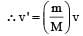Now, total K.E.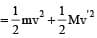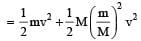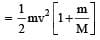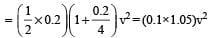But total K.E. = 1.05 kJ = 1.05 × 103J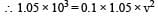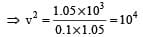∴ v = 102 = 100 ms–1.

31 Year NEET Previous Year Questions: Work, Energy & Power - 1 - Question 5

An engine pumps water continuously through a hose. Water leaves the hose with a velocity v and m is the mass per unit length of the water jet. What is the rate at which kinetic energy is imparted to water? 

Detailed Solution for 31 Year NEET Previous Year Questions: Work, Energy & Power - 1 - Question 5

m : mass per unit length

∴  rate of mass leaving the the hose per sec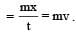Rate of K.E.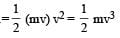31 Year NEET Previous Year Questions: Work, Energy & Power - 1 - Question 6

An explosion blows a rock into three parts. Two parts go off at right angles to each other. These two are, 1 kg first part moving with a velocity of 12 ms–1 and 2 kg second part moving with a velocity of 8 ms–1. If the third part flies off with a velocity of 4 ms–1, its mass would be: 

Detailed Solution for 31 Year NEET Previous Year Questions: Work, Energy & Power - 1 - Question 6

Let two parts of the rock move along x-axis and y-axis respectively.
If M and v be the mass and velocities of third part then
Mv cosθ = 12
Mv sinθ =16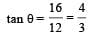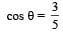v = 4 m/s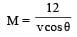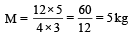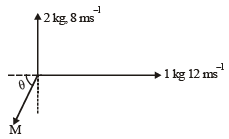31 Year NEET Previous Year Questions: Work, Energy & Power - 1 - Question 7

A body of mass 1 kg is thrown upwards with a velocity 20 m/s. It momentarily comes to rest after attaining a height of 18 m. How much energy is lost due to air friction? (g = 10 m/s2)

Detailed Solution for 31 Year NEET Previous Year Questions: Work, Energy & Power - 1 - Question 7

When the body is thrown upwards. its K.E is converted  into P.E. The loss of energy due to air friction is the difference of K.E and P.E.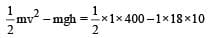= 200 – 180 = 20 J

31 Year NEET Previous Year Questions: Work, Energy & Power - 1 - Question 8

A ball moving with velocity 2 m/s collides head on with  another stationary ball of double the mass. If the coefficient of restitution is 0.5, then their velocities (in m/s) after  collision will be:

Detailed Solution for 31 Year NEET Previous Year Questions: Work, Energy & Power - 1 - Question 8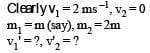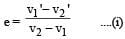By conservation of momentum, 2m = mv1' + 2mv2' ... (ii) From (i),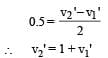From (ii), 2 = v1'+ 2 + 2 v1'

⇒  v1 = 0 and v2 = 1 ms–1

31 Year NEET Previous Year Questions: Work, Energy & Power - 1 - Question 9

An engine pumps water through a hose pipe.Water passes through the pipe and leaves it with a velocity of 2 m/s. The mass per unit length of water in the pipe is 100 kg/m. What is the power of the engine? 

Detailed Solution for 31 Year NEET Previous Year Questions: Work, Energy & Power - 1 - Question 9

Solution :- Amount of water flowing per second from the pipe

= m/time

= (m/l) *(l/t)

= (m/l)

Power = KE of water flowing per second

= 1/2(m/l)v.v^2

= 1/2(m/l)v3

= 12×100×8=400W

31 Year NEET Previous Year Questions: Work, Energy & Power - 1 - Question 10

The potential energy of a system increases if work is done 

Detailed Solution for 31 Year NEET Previous Year Questions: Work, Energy & Power - 1 - Question 10

The potential energy of a system increase if work is done by the system against a conservative force.
−ΔU = Wconservative force

31 Year NEET Previous Year Questions: Work, Energy & Power - 1 - Question 11

A body projected ver tically fr om the ear th reaches a height equal to earth's radius before returning to the earth. The power exerted by the gravitational force is greatest 

Detailed Solution for 31 Year NEET Previous Year Questions: Work, Energy & Power - 1 - Question 11

Power exerted by a force is given by P = F.v
When the body is just above the earth’s surface, its velocity is greatest. At this instant, gravitational force is also maximum.
Hence, the power exerted by the gravitational force is greatest at the instant just before the body hits the earth.

31 Year NEET Previous Year Questions: Work, Energy & Power - 1 - Question 12

Force F on a particle moving in a straight line varies with distance d as shown in the figure.The work done on the particle during its displacement of 12 m is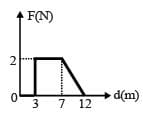Detailed Solution for 31 Year NEET Previous Year Questions: Work, Energy & Power - 1 - Question 12

Work done = area under F-d graph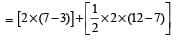= 8 + 5
= 13 J.

31 Year NEET Previous Year Questions: Work, Energy & Power - 1 - Question 13

A mass m moving horizontally (along the x-axis) with velocity v collides and sticks to mass of 3m moving vertically upward (along the y-axis) with velocity 2v. The final velocity of the combination is [2011M]

Detailed Solution for 31 Year NEET Previous Year Questions: Work, Energy & Power - 1 - Question 13

As the two masses stick together after collision, hence it is inelastic collision.
Therefore, only momentum is conserved.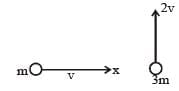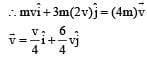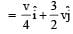31 Year NEET Previous Year Questions: Work, Energy & Power - 1 - Question 14

A solid cylinder of mass 3 kg is rolling on a horizontal surface with velocity 4 ms–1. It collides with a horizontal spring of force constant 200 Nm–1 . The maximum compression produced in the spring will be : 

Detailed Solution for 31 Year NEET Previous Year Questions: Work, Energy & Power - 1 - Question 14

At maximum compression the solid cylinder will stop so loss in K.E. of cylinder = gain in P.E. of spring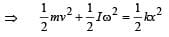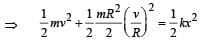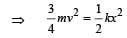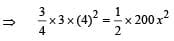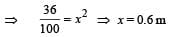31 Year NEET Previous Year Questions: Work, Energy & Power - 1 - Question 15

Two spheres A and B of masses m1 and m2 respectively collide. A is at rest initially and B is moving with velocity v along x-axis. After collision B has a velocity 2v  in a directionperpendicular to the original direction. The mass A moves after collision in the direction.

Detailed Solution for 31 Year NEET Previous Year Questions: Work, Energy & Power - 1 - Question 15

Solution :- Here initially sphere A is at rest and B is moving along x axis with velocity V.

After collision, velocity of sphere B becomes V/2 along direction perpendicular to its initial direction i.e. along Y axis.

Sphere A will move with some velocity u at some angle θ as shown in figure.considering law of conservation of momentum along X−axis.

m2V=m1ucosθ...........(i)

Considering law of conservation of momentum along Y−axis.

m2v/2=m1usinθ............(ii)

From equation i and ii.

1/2=tanθ

θ=tan−1(0.5).

31 Year NEET Previous Year Questions: Work, Energy & Power - 1 - Question 16

A car of mass m starts from rest and accelerates so that the instantaneous power delivered to the car has a constant magnitude P0. The instantaneous velocity of this car is proportional to : [2012M]

Detailed Solution for 31 Year NEET Previous Year Questions: Work, Energy & Power - 1 - Question 16

Constant power of car P0 = F.V = ma.v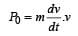P0dt = mvdv Integrating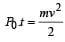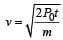∵  P0, m and 2 are constant31 Year NEET Previous Year Questions: Work, Energy & Power - 1 - Question 17

A uniform force of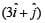newton acts on a particle of mass 2 kg. The particle is displaced from position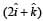meter to position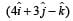meter. The work done by the force on the particle is [NEET 2013]

Detailed Solution for 31 Year NEET Previous Year Questions: Work, Energy & Power - 1 - Question 17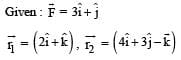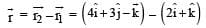So work done by the given force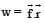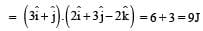31 Year NEET Previous Year Questions: Work, Energy & Power - 1 - Question 18

The upper half of an inclined plane of inclination θ is perfectly smooth while lower half is rough.
A block starting from rest at the top of the plane will again come to rest at the bottom, if the coefficient of friction between the block and lower half of the plane is given by [NEET 2013]

Detailed Solution for 31 Year NEET Previous Year Questions: Work, Energy & Power - 1 - Question 18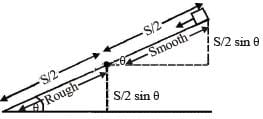For upper half of inclined  plane v2 = u2 + 2a S/2 = 2 (g sinθ) S/2 = gS sinθ
For lower half of inclined plane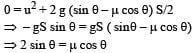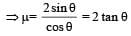31 Year NEET Previous Year Questions: Work, Energy & Power - 1 - Question 19

An explosion breaks a rock into three parts in a horizontal plane. Two of them go off at right angles to each other. The first part of mass 1 kg moves with a speed of 12 ms–1 and the second part of mass 2 kg moves with speed 8 ms–1. If the third part flies off with speed 4 ms–1 then its mass is [NEET 2013]

Detailed Solution for 31 Year NEET Previous Year Questions: Work, Energy & Power - 1 - Question 19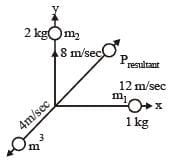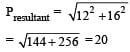m3v3 = 20 (momentum of third part)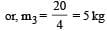31 Year NEET Previous Year Questions: Work, Energy & Power - 1 - Question 20

An explosion breaks a rock into three parts in a horizontal plane. Two of them go off at right angles to each other. The first part of mass 1 kg moves with a speed of 12 ms–1 and the second part of mass 2 kg moves with speed 8 ms–1. If the third part flies off with speed 4 ms–1 then its mass is [NEET 2013]

Detailed Solution for 31 Year NEET Previous Year Questions: Work, Energy & Power - 1 - Question 20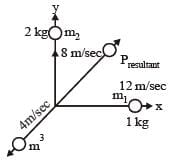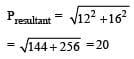m3v3 = 20 (momentum of third part)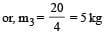31 Year NEET Previous Year Questions: Work, Energy & Power - 1 - Question 21

A person holding a rifle (mass of person and rifle together is 100 kg) stands on a smooth surface and fires 10 shots horizontally, in 5 s.Each bullet has a mass of 10 g with a muzzle velocity of 800 ms–1. The final velocity acquired by the person and the average force exerted on the person are [NEET Kar. 2013]

Detailed Solution for 31 Year NEET Previous Year Questions: Work, Energy & Power - 1 - Question 21

We have to use the Law of momentum conservation:

P ( initial ) = P ( final )

0 = n · m · u + ( M - n · m ) · v

where: n = 10, m = 10 g = 0.01 kg, u = 800 m/s, M = 100 kg.

0 = 10 · 0.01 kg · 800 m/s + ( 100 kg - 10 · 0.01 kg ) · v

v = - 80 kgm/s / 99.9 kgm/s

v = 0.8 m/s

Then : F = Δ P / Δ t = ( 10 · 0.01 kg · 800 m/s ) : 5 s = 16 N

Answer: The average force exerted on the person is 16 N.

31 Year NEET Previous Year Questions: Work, Energy & Power - 1 - Question 22

A particle with total energy E is moving in a potential energy region U(x). Motion of the particle is restricted to the region when [NEET Kar. 2013]

Detailed Solution for 31 Year NEET Previous Year Questions: Work, Energy & Power - 1 - Question 22

As the particle is moving in a potential energy region.

∴ Kinetic energy ≥ 0 And, total energy E = K.E. + P.E.

⇒ U(x) ≤ E

31 Year NEET Previous Year Questions: Work, Energy & Power - 1 - Question 23

One coolie takes 1 minute to raise a suitcase through a height of 2 m but the second coolie takes 30 s to raise the same suitcase to the same height. The powers of two coolies are in the ratio of [NEET Kar. 2013]

Detailed Solution for 31 Year NEET Previous Year Questions: Work, Energy & Power - 1 - Question 23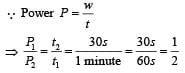(t1 = 1 minute; t2 = 30 second given)

## Physics Class 11

127 videos|464 docs|210 tests
 Use Code STAYHOME200 and get INR 200 additional OFF Use Coupon Code
Information about 31 Year NEET Previous Year Questions: Work, Energy & Power - 1 Page
In this test you can find the Exam questions for 31 Year NEET Previous Year Questions: Work, Energy & Power - 1 solved & explained in the simplest way possible. Besides giving Questions and answers for 31 Year NEET Previous Year Questions: Work, Energy & Power - 1, EduRev gives you an ample number of Online tests for practice

## Physics Class 11

127 videos|464 docs|210 tests

### How to Prepare for NEET

Read our guide to prepare for NEET which is created by Toppers & the best Teachers